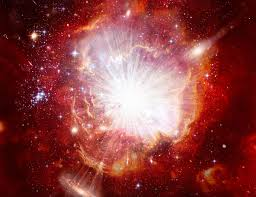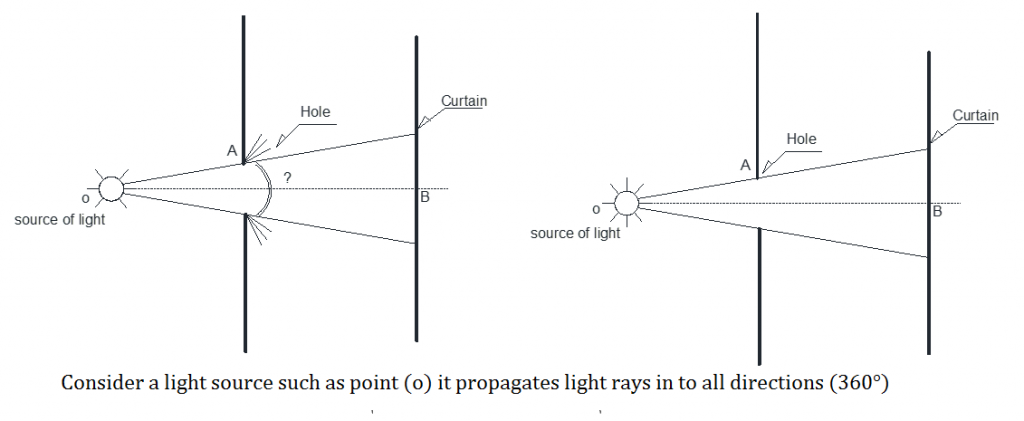Select Page# Light propagation (Big Bang)

#### Physics , Mechanic , Mathematic

Light propagation (Big Bang)

There are 2 discussions in the phenome none of light propagation:

1. Geometric optics in which only light rays are considered.
2. Wave optics in which the wave nature of light receives attention.

In the presented paper, our discussion is on geometric optics. In light physics, light speed has been measured and regarding the conducted experiment, light speed has been measured to be (V=300,000 km/sec)

Let’s consider a lamp in 2 states:

1. Lamp in the OFF state.
2. Lamp in the ON state.
1. In this state, the lamp being OFF, no ray comes out of the light source (lamp). That is, light speed in this state equals zero.
2. In this state, switching the lamp on will turn it on. Light speed will thus equal propagation.

A remarkable point: (V=300,000 km/sec)

In order for light speed to reach from state (A) to state (B), light speed should increase from zero to (V=300,000 km/sec)

Therefore, in order for light speed to get from zero to (V=300,000 km/sec) or from state (A) to state (B), a time interval will be required between the 2 states:

The following experiment is performed to obtain the interval between states (A) and (B), named in physics course books as “light refraction point” , “light deviation point” or ….